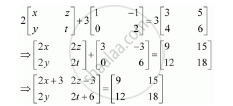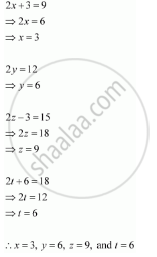Share

# Solve the Equation for X, Y, Z and T If 2 Matrice[(X,Z),(Y, T)] + 3 Matrice[(1,-1),(0,2)] = 3[(3,5),(4,6)] - CBSE (Science) Class 12 - Mathematics

ConceptOperations on Matrices Properties of Scalar Multiplication of a Matrix

#### Question

Solve the equation for x, y, z and t if 2[(x,z),(y, t)] + 3[(1,-1),(0,2)] = 3[(3,5),(4,6)]

#### SolutionComparing the corresponding elements of these two matrices, we get:Is there an error in this question or solution?

#### APPEARS IN

Solution Solve the Equation for X, Y, Z and T If 2 Matrice[(X,Z),(Y, T)] + 3 Matrice[(1,-1),(0,2)] = 3[(3,5),(4,6)] Concept: Operations on Matrices - Properties of Scalar Multiplication of a Matrix.
S